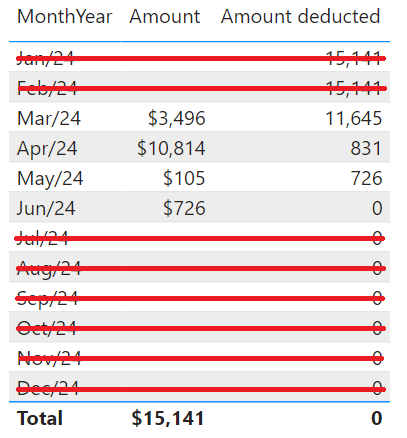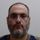cancel
Showing results for
Did you mean:

Fabric is Generally Available. Browse Fabric Presentations. Work towards your Fabric certification with the Cloud Skills Challenge.Resolver I

## Showing ALL() values - but only when there are values in another columns

Hey there

Is there any way to show the rows in the following table but only when there is a value for the month in the filter context?The measure that calculates the Amount deducted is the following:

``````Amount deducted =
VAR _ALL = CALCULATE( [Amount] , ALL( d_calendar ) ) -- , CALCULATETABLE( Example ) )
VAR _Result =
CALCULATE(
[Amount],
FILTER(
ALL( d_calendar ),
d_calendar[Date] <= MAX( d_calendar[Date] )
)
)
RETURN
_ALL - _Result``````

I've even tried to do that by using CALCULATETABLE() inside the CALCULATE statement, but it has not properly worked.

Do any of you know how to blank out all the red-outlined lines of the image? Something like that is expected:1 ACCEPTED SOLUTIONSuper User

pls try this

``````Amount deducted =
VAR _ALL = CALCULATE( [Amount] , ALL( d_calendar ) ) -- , CALCULATETABLE( Example ) )
VAR _Result =
CALCULATE(
[Amount],
FILTER(
ALL( d_calendar ),
d_calendar[Date] <= MAX( d_calendar[Date] )
)
)
RETURN
IF([Amount],_ALL - _Result)``````
2 REPLIES 2Super User

pls try this

``````Amount deducted =
VAR _ALL = CALCULATE( [Amount] , ALL( d_calendar ) ) -- , CALCULATETABLE( Example ) )
VAR _Result =
CALCULATE(
[Amount],
FILTER(
ALL( d_calendar ),
d_calendar[Date] <= MAX( d_calendar[Date] )
)
)
RETURN
IF([Amount],_ALL - _Result)``````Resolver I

Can't believe the solution is that simple, my friend. Thank you very much.

I've been trying to fix that all the week, but always got the CallBackDataId, which screw the performance in my real-life report. However, with your piece of code you managed to help me out. Cant say other thing but thanks.

Have a nice day.Announcements#### Power BI Monthly Update - November 2023

Check out the November 2023 Power BI update to learn about new features.#### The largest Power BI and Fabric virtual conference

130+ sessions, 130+ speakers, Product managers, MVPs, and experts. All about Power BI and Fabric. Attend online or watch the recordings.Top Solution Authors
Top Kudoed Authors
Users online (5,937)